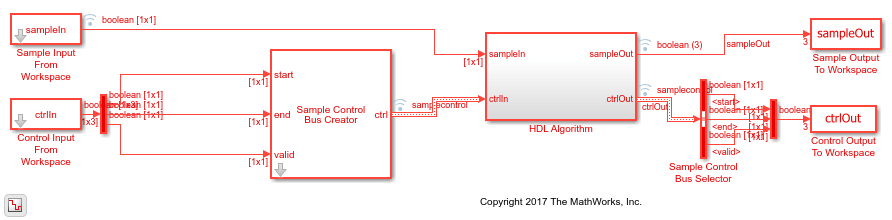# Convolutional Encode of Streaming Samples

This example shows how to use the LTE Convolutional Encoder block to encode data, and how to compare the hardware-friendly design with the results from LTE Toolbox™. The workflow follows these steps:

1. Generate frames of random input samples in MATLAB®.

2. Encode the data using the LTE Toolbox function `lteConvolutionalEncode`.

3. Convert framed input data to a stream of samples and import the stream into Simulink®.

4. To encode the samples using a hardware-friendly architecture, run the Simulink model, which contains the Wireless HDL Toolbox™ block LTE Convolutional Encoder.

5. Export the stream of encoded bits to the MATLAB workspace.

6. Convert the sample stream back to framed data, and compare the frames with the reference data.

Generate input data frames. Generate reference encoded data using `lteConvolutionalEncode`.

```rng(0); frameLength = 256; numframes = 2; txframes = cell(1,numframes); txcodeword = cell(1,numframes); rxSoftframes = cell(1,numframes); for k = 1:numframes txframes{k} = randi([0 1],frameLength,1)>0.5; txcodeword{k} = lteConvolutionalEncode(txframes{k}); end ```

Serialize input data for the Simulink model. Leave enough time between frames so that each frame is fully encoded before the next one starts. The block takes `frameLength` + 5 cycles to encode the frame.

```idleCyclesBetweenSamples = 0; idleCyclesBetweenFrames = frameLength+5; [sampleIn,ctrlIn] = whdlFramesToSamples(... txframes,idleCyclesBetweenSamples,idleCyclesBetweenFrames); ```

Run the Simulink model. Because of the added idle cycles between frames, the streaming input data includes enough cycles for the model to complete encoding of both frames.

```sampletime = 1; samplesizeIn = 1; simTime = size(ctrlIn,1); modelname = 'ltehdlConvolutionalEncoderModel'; open_system(modelname); sim(modelname); ```The Simulink model exports `sampleOut` and `ctrlOut` back to the MATLAB workspace. Deserialize the output samples, and compare them to the encoded frame.

The output samples of the LTE Convolutional Encoder block are the interleaved results of the three polynomials.

• Hardware-friendly output: `G0_1 G1_1 G2_1 G0_2 G1_2 G2_2 ... Gn G1_n G2_n`

• LTE Toolbox output: `G0_1 G0_2 ... G0_n G1_1 G1_2 ... G1_n G2_1 G2_2 ... G2_n`

The `whdlSamplesToFrames` function provides an option to reorder the samples. Compare the reordered output frames with the reference encoded frames.

```interleaveSamples = true; sampleOut = sampleOut'; txhdlframes = whdlSamplesToFrames(sampleOut(:),ctrlOut,[],interleaveSamples); fprintf('\nLTE Convolutional Encoder\n'); for k = 1:numframes numBitsDiff = sum(double(txcodeword{k})-double(txhdlframes{k})); fprintf([' Frame %d: Behavioral and ' ... 'HDL simulation differ by %d bits\n'],k,numBitsDiff); end ```
```Maximum frame size computed to be 768 samples. LTE Convolutional Encoder Frame 1: Behavioral and HDL simulation differ by 0 bits Frame 2: Behavioral and HDL simulation differ by 0 bits ```